## Multiply Excited Magnetic Field System:

Multiply Excited Magnetic Field System – Singly-excited devices discussed earlier, are generally employed for motion through a limited distance or rotation through a prescribed angle.

Electro-mechanical transducers have the special requirement of producing an electrical signal proportional to forces or velocities or producing force proportional to electrical signal (current or voltage). Such transducers require two excitation one excitation establishes a magnetic field of specified strength while the other excitation produces the desired signal (electrical or mechanical).

Also continuous energy conversion devices motors and generators require multiple excitation.

Figure 4.15 shows a magnetic field system with two electrical excitations one on stator and the other on rotor. The system can be described in either of the two sets of three independent variables; (λ1, λ2, θ) or (i1, i2, θ). In terms of the first set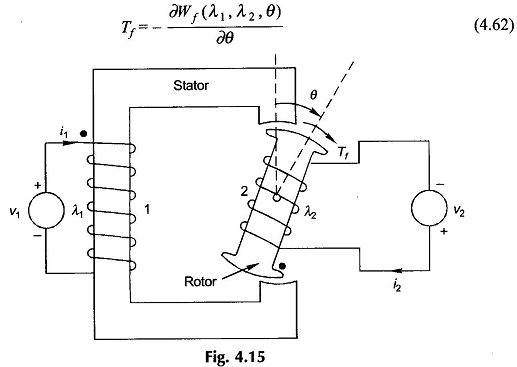where the field energy is given by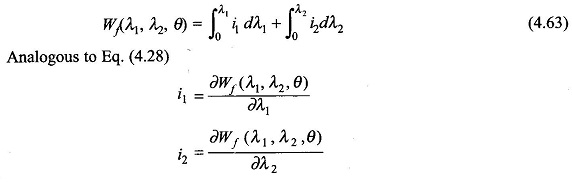Assuming linearity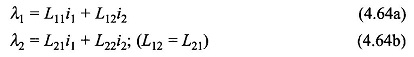Solving for i1 and i2 in terms of λ1, λ2 and substituting in Eq. (4.63) gives upon integration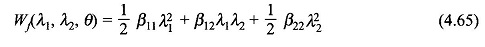where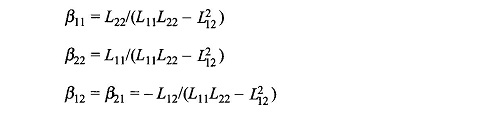The Self- and mutual-inductance of the two exciting coils are functions are angle θ.

If currents are used to describe the system state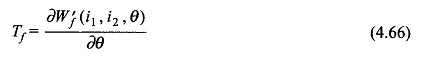where the coenergy is given by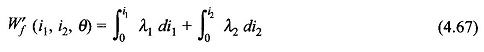In the linear case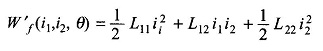where inductances are functions of angle θ.

Scroll to Top